# Image Processing 101: Image Enhancement

## What is Image Enhancement?

Image enhancement refers to the process of highlighting certain information of an image, as well as weakening or removing any unnecessary information according to specific needs. For example, eliminating noise, revealing blurred details, and adjusting levels to highlight features of an image.

Image enhancement techniques can be divided into two broad categories:

• Spatial domain — enhancement the image space that divides an image into uniform pixels according to the spatial coordinates with a certain resolution. The spatial domain methods perform operations on pixels directly
• Frequency domain — enhancement obtained by applying the Fourier Transform to the spatial domain. In the frequency domain, pixels are operated in groups as well as indirectly.

## Image Enhancement Techniques

This section discusses the image enhancement techniques implemented in the spatial domain. The concept is to map every pixel onto a new image with a predefined transformation function.

g(x, y) = T(f(x, y))

• g (x, y) is the output image
• T is an operator
• f (x, y) is the input image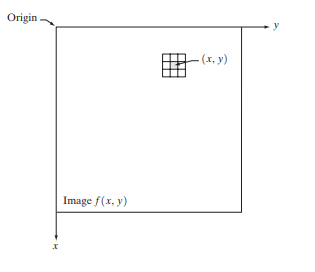Image source: Slideshare.net

The figure shows a 3 x 3 neighborhood (or spatial filter) of the point (x, y) in an image spatial domain. Moving the neighborhood from pixel to pixel (a procedure called spatial filtering) can generate a new image.

## Basic Intensity Transformation Functions

The simplest image enhancement method is to use a 1 x 1 neighborhood size. In this case, the output pixel only depends on the input pixel and the function can be simplified as:

s = T(r)

Different transformation functions work for different scenarios.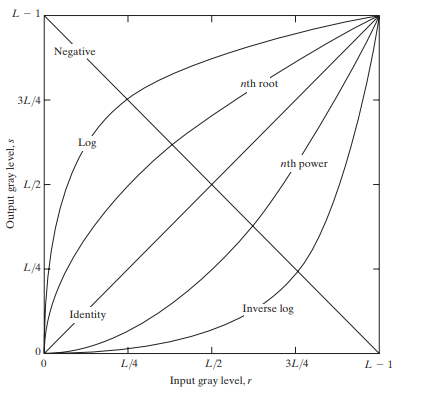Image source: Slideshare.net

### Linear

Linear transformations include identity transformation and negative transformation.

In identity transformation, the input image is the same as the output image.

s = r

The negative transformation is:

s = L – 1 – r = 256 – 1 – r = 255 – r

This kind of transformation is suited for enhancing white or gray detail embedded in dark areas of an image. For example, analyzing the breast tissue in a digital mammogram.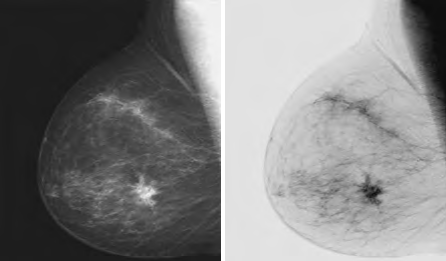Image source: Slideshare.net

### Logarithmic

The equation of general log transformation is:

s = clog(1 + r)

• c is a constant

In the log transformation, the low intensity values are mapped into higher intensity values.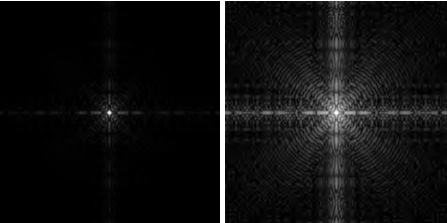Image source: Slideshare.net

The inverse log transform is opposite to log transform.

### Power-Law

Power-law transformation equation is: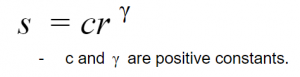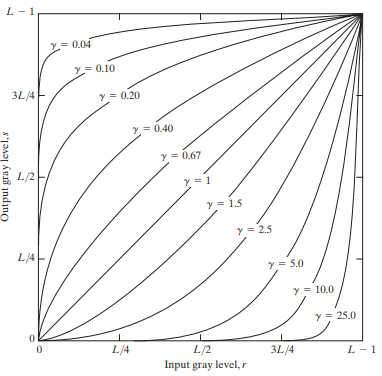Image source: Slideshare.net

Comparing to log transformation, gamma transformation can generate a family of possible transformation curves by varying the gamma value.

Here are the enhanced images output by using different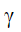values.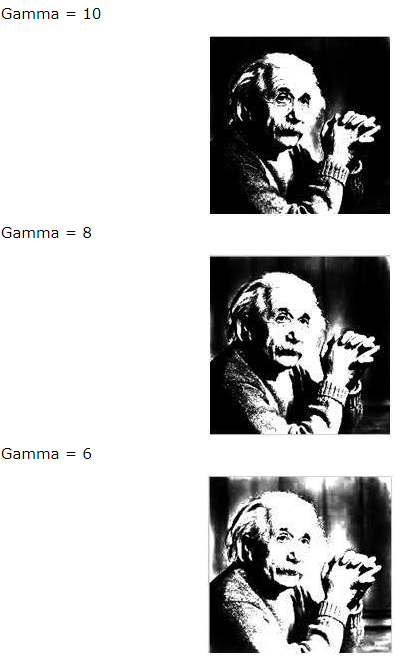Image source: TutorialsPoint.com

### Histogram Equalization

This method is to boost the global contrast of an image to make it look more visible.

The general histogram equalization formula is: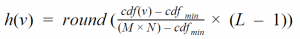• CDF refers to the cumulative distribution function
• L is the maximum intensity value (typically 256)
• M is the image width and N is the image height
• h (v) is the equalized value

Image source: Wikipedia

Image source: Wikipedia